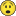# QlikView App Dev

Discussion Board for collaboration related to QlikView App Development.

Announcements
Read about the latest Qlik Community enhancements on the Community News blog!
cancel
Showing results for
Did you mean:Creator III

## Rangeavg bug with zero values?

Hi I have a pivot table where I'm using rangeavg +  agg to calculate the last 3 months average for current month. It works like a charm when there are no zeros in any of the months, but when there are, the result is 0 even when there's data for the other 2 months.

Do you know the solution to this?

1 Solution

Accepted SolutionsMVP

See if one of these work for you

RangeAvg(After(TOTAL Sum({<MONTH1=,MONTH=,MONTH_NAME=,YEAR=,QUARTER=,Q=, SUBJECT={'DELIVER'}>} LN_COUNT),0,3))

or

RangeAvg(After(TOTAL Sum({<MONTH1=,MONTH=,MONTH_NAME=,YEAR=,QUARTER=,Q=>} IF(SUBJECT='DELIVER', LN_COUNT,0)),0,3))

8 RepliesMVP

May be do RangeSum() and divide by 3?Creator III
Author

I tried that, but rangesum is returning 0 as well.....

sum(aggr(Rangesum(above(sum({<MONTH1=,MONTH=,MONTH_NAME=,YEAR=,QUARTER=,Q=>}IF(SUBJECT='DELIVER', LN_COUNT,0)),0,3)),CUSTOMER_CHANNEL_GROUP,CUST_RTM_GROUP,SEGMENT,PODI_GROUPED,PLANNING_STRATEGIC_REGION_CD,MONTH))/3MVP

Would you be able to share an example where it isn't working?Creator III
Author

Here's the dashboard I have, in the container is the tab "Order", column "Den" (this is the one that calcualtes the 3 months avg)

The data for the average is the one in the tab "Delivery" column "Den"

I have 1 big fact table with a "Subject" table.

I actually noticed that there's no "DELIVER" data in Sept for MCA, this is causing the 0....

Any Idea how to fix it?MVP

Where do I see MCA? I am hard time finding it in the sample. Apologize if it is right thereCreator III
Author

No worries, I didn't make myself clear. Expand the last dimension column to see it... I just ran a dummy example with an inline load..... so it won't calculate unless  at least one expression has data for Sep... in this particular neither Num nor Den has data for Sep.... I will have to generate dummy data for each record at its lowest level I guessany idea how to easily solve this?MVP

See if one of these work for you

RangeAvg(After(TOTAL Sum({<MONTH1=,MONTH=,MONTH_NAME=,YEAR=,QUARTER=,Q=, SUBJECT={'DELIVER'}>} LN_COUNT),0,3))

or

RangeAvg(After(TOTAL Sum({<MONTH1=,MONTH=,MONTH_NAME=,YEAR=,QUARTER=,Q=>} IF(SUBJECT='DELIVER', LN_COUNT,0)),0,3))Creator III
Author

Thank you very much Sir. I didn't know this could be done without using aggrCommunity Browser